## Plotting in R

The first data set, Nursing, from the Stat2Data textbook, includes characteristics obtained from a nursing home in New Mexico.

Here is a description of the variables in the data set:

Variables Descriptions
Beds Number of beds in the nursing home
InPatientDays Annual medical in-patient days (in hundreds)
AllPatientDays Annual total patient days (in hundreds)
PatientRevenue Annual patient care revenue (in hundreds of dollars)
NurseSalaries Annual nursing salaries (in hundreds of dollars)
FacilitiesExpend Annual facilities expenditure (in hundreds of dollars)
Rural 1=rural or 0=non-rural

*Note: the \(\texttt{NurseSalaries}\) variable is the total salary per year for ALL nurses.

Let’s say we are interested in if the annual salaries of all the nurses at a nursing facility are inversely related to the number of annual in-patient days. It seems plausible that nurses who were paid better may be more experienced and satisfied with their job and therefore could help patients recover more quickly. Clearly this is not the only variable that should be included. Think about what other variables might help explain the annual medical in-patient days.

``````## Load the data from Stat2Data
data("Nursing")``````

\(\texttt{ggplot2}\) is a package in R that allows R users to create elegant data visualizations. There are many commands that allow you to customize your plots including color, background, text size, labeling, etc. Nearly all plots can also be done using base R functions, but nothing really looks a nice as ggplot.

``````# Make sure you install the library: install.packages("ggplot2")
library(ggplot2)``````

### Exploratory Data Analysis

To get an idea of what a data set looks like, statisticians perform what we call an Exploratory Data Analysis (EDA).

``````#### Summary statistics
summary(Nursing[, c(2, 5)])``````
``````##  InPatientDays   NurseSalaries
##  Min.   : 48.0   Min.   :1288
##  1st Qu.:125.2   1st Qu.:2336
##  Median :164.5   Median :3696
##  Mean   :183.9   Mean   :3813
##  3rd Qu.:229.0   3rd Qu.:4840
##  Max.   :514.0   Max.   :7489``````
``````#### Check for outliers in x and y
# Histogram using base R libraries

hist(Nursing\$NurseSalaries)``````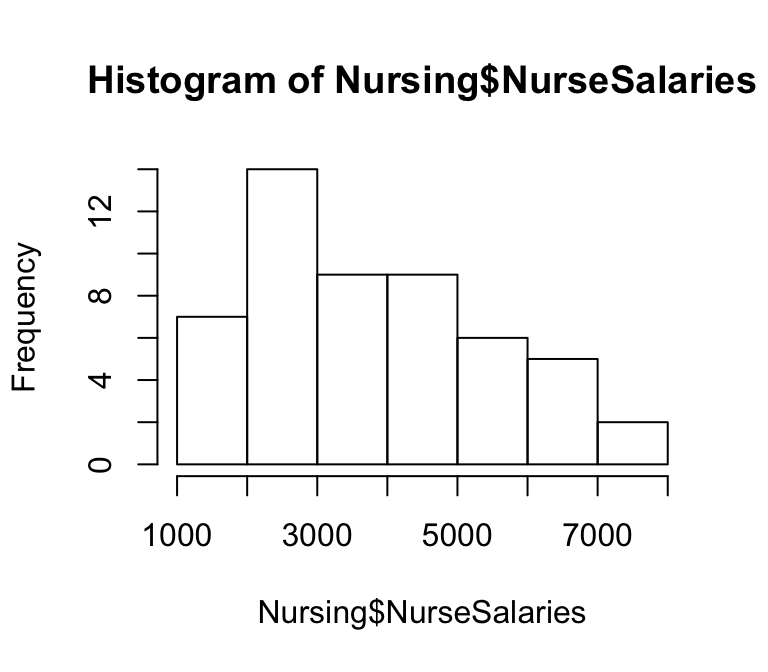``hist(Nursing\$InPatientDays) # Looks like there is one extreme outlier``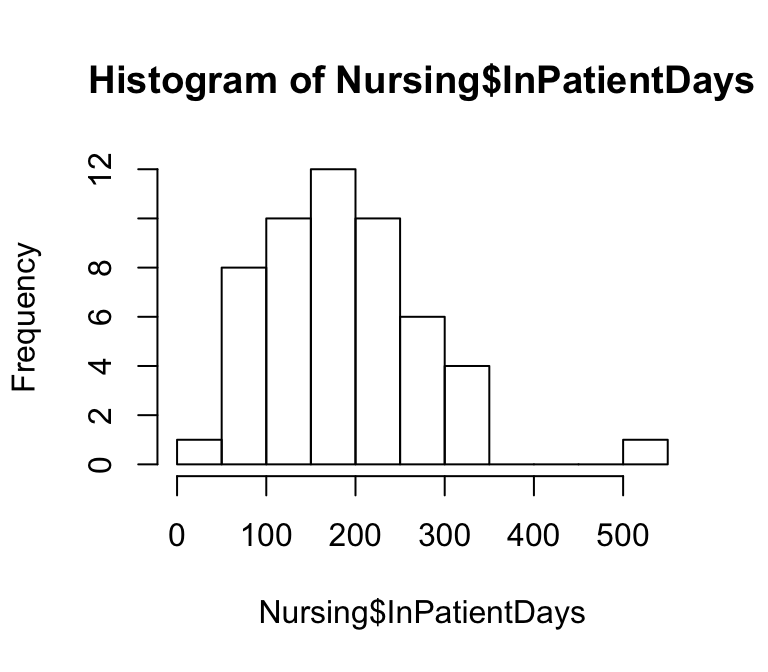``````# Histogram using ggplot2
ggplot(Nursing) + geom_histogram(aes(x = NurseSalaries)) # binwidth is weird``````
``## `stat_bin()` using `bins = 30`. Pick better value with `binwidth`.``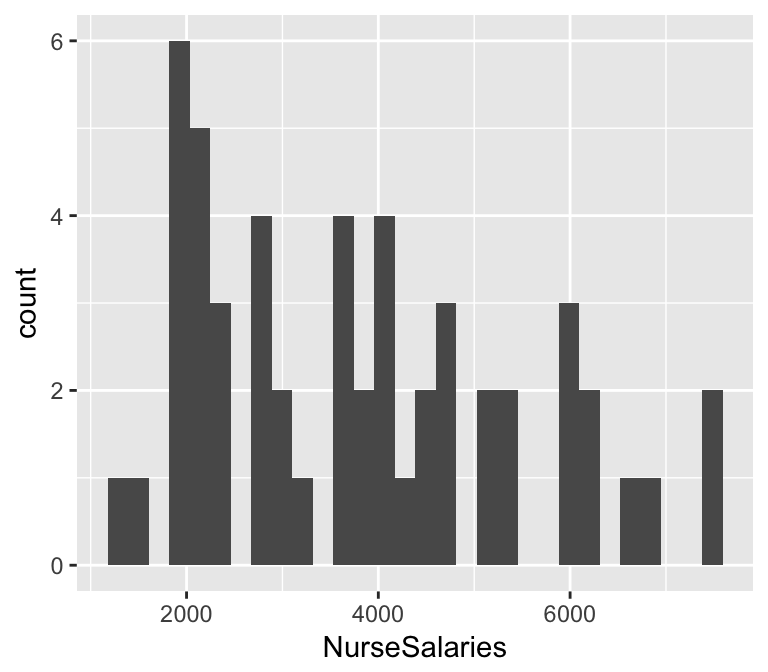``ggplot(Nursing) + geom_histogram(aes(x = NurseSalaries), binwidth = 750) # better binwidth``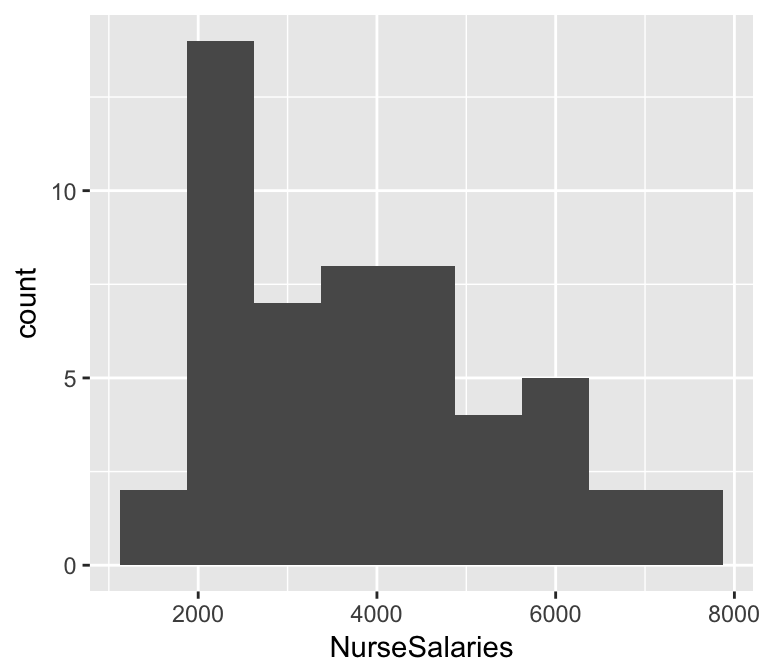``````#### How does the number of in-patient days change depending on the environment?
## Use a boxplot!

# First, we need to make "Rural" a factor
Nursing\$Rural <- factor(Nursing\$Rural, levels = c(0,1), labels = c("Urban", "Rural"))
ggplot(Nursing) + geom_boxplot(aes(x = Rural, y = InPatientDays))``````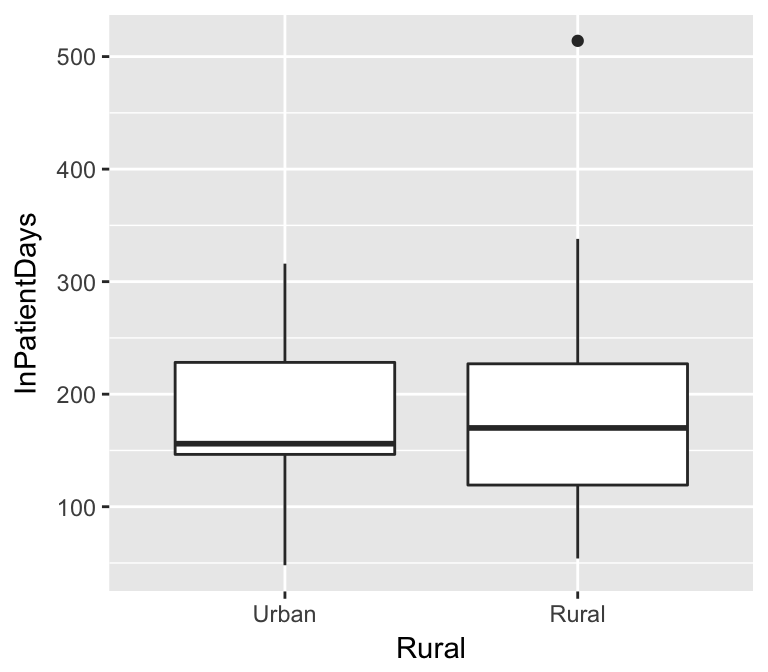### Prediction

Now to the modeling. To determine if it makes sense to model the relationship between the number of annual in-patient days and nurse salaries linearly, we should use a scatterplot.

``````# (Well labeled) scatterplot using base R functions
plot(InPatientDays ~ NurseSalaries, data = Nursing,
main = "In-Patient Days vs Nurse Salaries",
xlab = "Nurse Salaries (in 100's of dollars)",
ylab = "Annual In-Patient Days (in 100's)")``````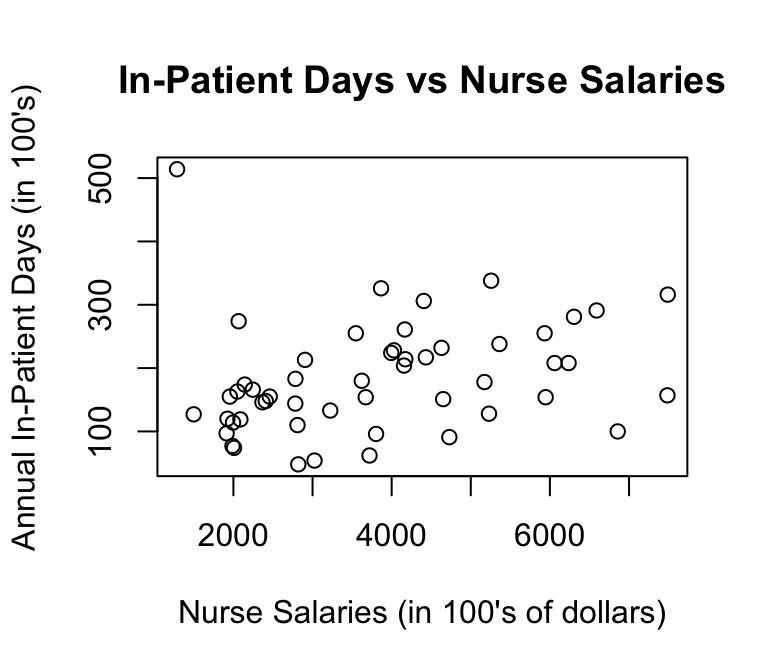``````# (Well labeled) scatterplot using ggplot2
ggplot(Nursing) + geom_point(aes(x = NurseSalaries, y = InPatientDays)) +
ggtitle("Scatterplot: In-Patient Days against Nurse Salaries") +
xlab("Nurse Salaries (in 100's of dollars)") +
ylab("Annual In-Patient Days (in 100's)")``````